# E-PolyLearning

 11. Thermal diffusivity is given by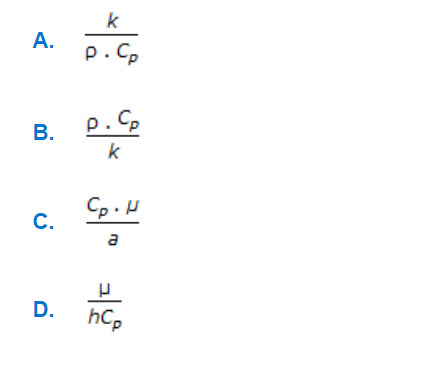a. A b. B c. C d. D

 12. At steady state the temperature variation in a plane wall, made of two different solids I & II is shown below. The thermal conductivity of material I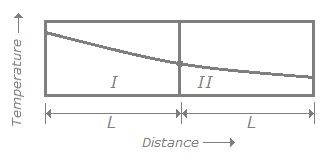a. is smaller than that of II. b. is greater than that of II. c. is equal to that of II. d. can be greater than or smaller than that of II.
 13. In case of surface condensers, a straight line is obtained on plotting 1/U vs __________ on an ordinary graph paper.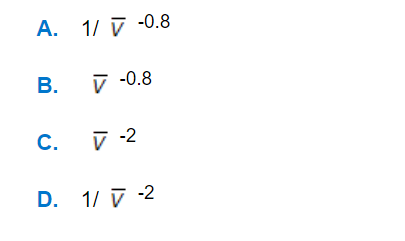a. A b. B c. C d. D
 14. Fouling factor for a heat exchanger is given by (where, U₁ = heat transfer co-efficient of dirty surface U₂ = heat transfer co-efficient of clean surface)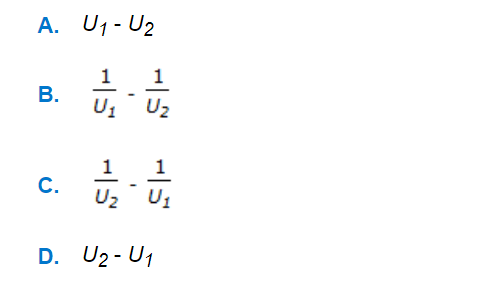a. A b. B c. C d. D
 15. For a counter current heat exchanger with Tih = 80°C, T°c = 60°C, T°h = 50°C and Tic = 30°C, and the temperature difference between the two streams being the same everywhere along Z, the direction of flow of hot fluid. The temperature profile should satisfy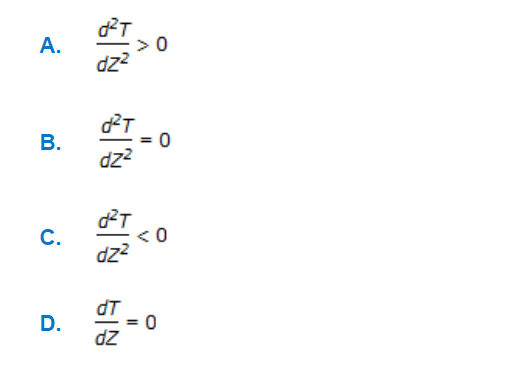a. A b. B c. C d. D